# imsegfmm

Binary image segmentation using fast marching method

## Syntax

``BW = imsegfmm(W,mask,thresh)``
``BW = imsegfmm(W,C,R,thresh)``
``BW = imsegfmm(W,C,R,P,thresh)``
``````[BW,D] = imsegfmm(___)``````

## Description

example

````BW = imsegfmm(W,mask,thresh)` returns a segmented image `BW`, which is computed using the fast marching method. The array `W` specifies weights for each pixel. `mask` is a logical array that specifies seed locations. `thresh` specifies the threshold level.```
````BW = imsegfmm(W,C,R,thresh)` returns a segmented image, with seed locations specified by the vectors `C` and `R`, which contain column and row indices. `C` and `R` must contain values which are valid pixel indices in `W`.```
````BW = imsegfmm(W,C,R,P,thresh)` returns a segmented image, with seed locations specified by the vectors `C`, `R`, and `P`, which contain column, row, and plane indices. `C`, `R`, and `P` must contain values which are valid pixel indices in `W`.```
``````[BW,D] = imsegfmm(___)``` returns the normalized geodesic distance map `D` computed using the fast marching method. `BW` is a thresholded version of `D`, where all the pixels that have normalized geodesic distance values less than `thresh` are considered foreground pixels and set to `true`. `D` can be thresholded at different levels to obtain different segmentation results.```

## Examples

collapse all

This example shows how to segment an object in an image using Fast Marching Method based on differences in grayscale intensity as compared to the seed locations.

```I = imread('cameraman.tif'); imshow(I) title('Original Image')```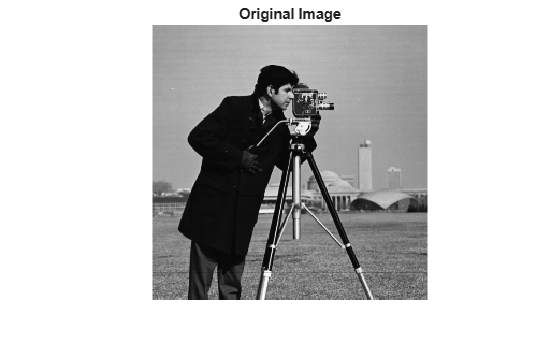Create mask and specify seed location. You can also use `roipoly` to create the mask interactively.

```mask = false(size(I)); mask(170,70) = true;```

Compute the weight array based on grayscale intensity differences.

`W = graydiffweight(I, mask, 'GrayDifferenceCutoff', 25);`

Segment the image using the weights.

```thresh = 0.01; [BW, D] = imsegfmm(W, mask, thresh); figure imshow(BW) title('Segmented Image')```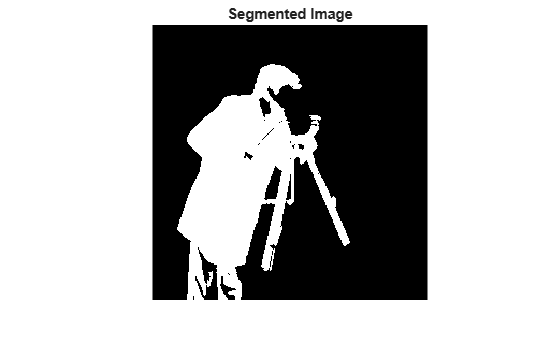You can threshold the geodesic distance matrix `D` using different thresholds to get different segmentation results.

```figure imshow(D) title('Geodesic Distances')```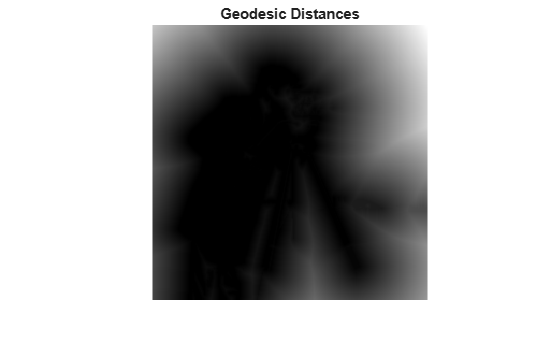This example segments the brain from MRI data of the human head.

```load("mri") V = squeeze(D);```

Visualize the data.

```sizeO = size(V); figure slice(double(V),sizeO(2)/2,sizeO(1)/2,sizeO(3)/2); shading interp colormap("gray") title("Original")```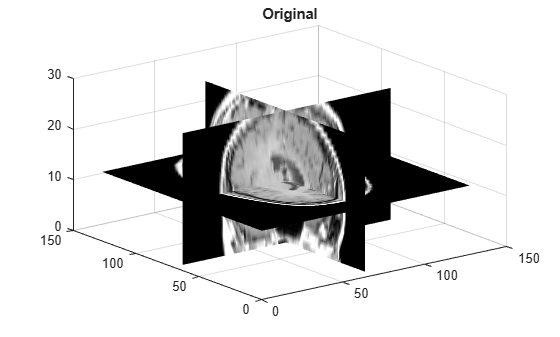Set the seed locations.

```seedR = 75; seedC = 60; seedP = 10;```

Compute weights based on grayscale intensity differences.

`W = graydiffweight(V,seedC,seedR,seedP,"GrayDifferenceCutoff",25);`

Segment the image using the weights.

```thresh = 0.002; BW = imsegfmm(W,seedC,seedR,seedP,thresh);```

Visualize the segmented image using an isosurface.

```figure p = patch(isosurface(double(BW))); p.FaceColor = "red"; p.EdgeColor = "none"; daspect([1 1 27/64]); camlight lighting phong```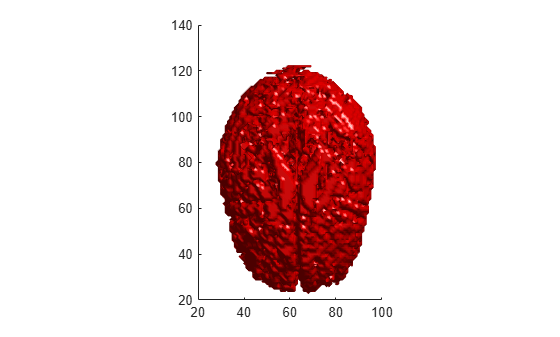## Input Arguments

collapse all

Weight array, specified as a non-negative numeric array. You can compute the weight array by using the `graydiffweight` or `gradientweight` functions. Large values in `W` identify the foreground (object) and small values identify the background.

Data Types: `single` | `double` | `uint8` | `int8` | `int16` | `uint16` | `int32` | `uint32`

Seed locations mask, specified as a logical array of the same size as `W`. Locations where `mask` is `true` are seed locations. If you use `graydiffweight` to create the weight matrix `W`, it is recommended that you use the same value of `mask` with `imsegfmm` that you used with `graydiffweight`.

Data Types: `logical`

Threshold level used to obtain the binary image, specified as a number in the range [0, 1]. Low values typically result in large foreground regions (logical true) in `BW`, and high values produce small foreground regions.

Example: `0.5`

Data Types: `double`

Column index of reference pixels, specified as a numeric vector.

Example: `[50 75 93]`

Data Types: `double`

Row index of reference pixels, specified as a numeric vector.

Example: `[48 71 89]`

Data Types: `double`

Plane index of reference pixels, specified as a numeric vector.

Example: `[2 4 7]`

Data Types: `double`

## Output Arguments

collapse all

Segmented image, returned as a logical array of the same size as `W`.

Data Types: `logical`

Normalized geodesic distance map, returned as a numeric array of the same size as `W`. If `W` is of class `single`, then `D` is of class `single`. Otherwise, `D` is of class `double`.

Data Types: `double` | `single`

## Tips

• `imsegfmm` uses double-precision floating point operations for internal computations for all classes except class `single`. If `W` is of class `single`, `imsegfmm` uses single-precision floating point operations internally.

• `imsegfmm` sets pixels with `0` or `NaN` weight values to `Inf` in the geodesic distance image `D`. These pixels are part of the background (logical false) in the segmented image `BW`.

 Sethian, J. A. Level Set Methods and Fast Marching Methods: Evolving Interfaces in Computational Geometry, Fluid Mechanics, Computer Vision, and Materials Science, Cambridge University Press, 2nd Edition, 1999.